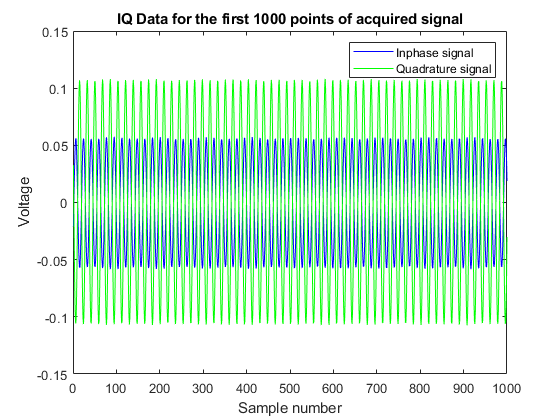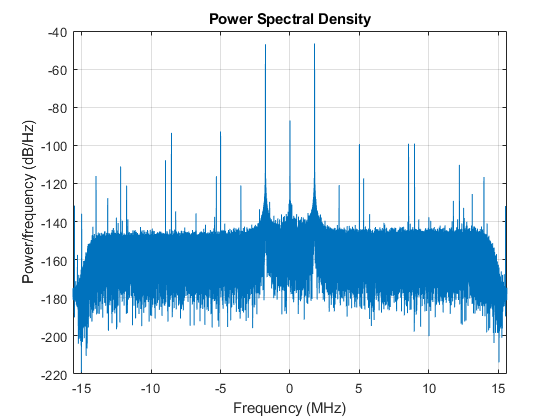# Reading Inphase and Quadrature (IQ) Data from a Signal Analyzer over TCP/IP

This example shows how to acquire IQ Data from a signal analyzer over a TCP/IP interface.

Instrument Control Toolbox™ supports communication with instruments through interfaces and drivers.

For a complete list of supported hardware, visit the Instrument Control Toolbox product page.

### Introduction

This example acquires IQ Data from a Keysight Technologies® (formerly Agilent Technologies® ) X-Series Signal Analyzer (N9030A, PXA Signal Analyzer) over a TCP/IP interface.

### Requirements

To run this example you need an X-Series Signal analyzer with an Ethernet (TCP/IP) connection. You can also execute this example with MATLAB on your X-Series analyzer, or on a PC on the same network as the X-Series Analyzer.

This example uses functions from the Instrument Control Toolbox and the DSP System Toolbox™.

### Define Measurement Parameters

Define the parameters used to configure the instrument before you make the measurement. Based on the signal you are measuring, you may need to modify some of the following parameters.

```% Specify the IP address of the signal analyzer addressMXA = "172.28.16.61"; ```

### Parameter Definitions

```% Center frequency of the modulated waveform (Hz) centerFrequency = 2.14e9; % Bandwidth of the signal (Hz) bandwidth = 25e6; % Measurement time (s) measureTime = 8e-3; % Mechanical attenuation in the signal analyzer(dB) mechAttenuation = 0; % Start frequency for Spectrum Analyzer view startFrequency = 2.11e9; % Stop frequency for Spectrum Analyzer view stopFrequency = 2.17e9; % Resolution Bandwidth for Spectrum Analyzer view resolutionBandwidth = 200e3; % Video Bandwidth for Spectrum Analyzer view videoBandwidth = 300; ```

### Connect to the Instrument

• Set up instrument connectivity using a TCP/IP connection.

• Set the timeout to allow sufficient time for the measurement and transfer of data.

• Set the byte order to be "big-endian" to read the floating point data in the correct format from the analyzer.

```signalAnalyzerObject = tcpclient(addressMXA, 5025); signalAnalyzerObject.ByteOrder = "big-endian"; signalAnalyzerObject.Timeout = 20; ```

### Query Instrument Identification Information

Reset the instrument to a known state using the appropriate SCPI command. Query the instrument identity to make sure we are connected to the right instrument.

```writeline(signalAnalyzerObject, "*RST"); instrumentInfo = writeread(signalAnalyzerObject, "*IDN?"); disp("Instrument identification information: " + instrumentInfo); ```
```Instrument identification information: Agilent Technologies,N9030A,US00071181,A.14.16 ```

### Set Up Instrument for an IQ Waveform Measurement

The X-Series signal and spectrum analyzers perform IQ measurements as well as spectrum measurements. In this example, you acquire the time domain IQ data, visualize it in MATLAB, and perform signal analysis on the acquired data. Use SCPI commands to configure the instrument to make the measurement and define the format of the data transfer once the measurement is made.

```% Set up signal analyzer mode to Basic/IQ mode writeline(signalAnalyzerObject,":INSTrument:SELect BASIC"); % Set the center frequency writeline(signalAnalyzerObject,":SENSe:FREQuency:CENTer " + num2str(centerFrequency)); % Set the resolution bandwidth writeline(signalAnalyzerObject,":SENSe:WAVEform:BANDwidth:RESolution " + num2str(bandwidth)); % Turn off averaging writeline(signalAnalyzerObject,":SENSe:WAVeform:AVER OFF"); % Set to take one single measurement once the trigger line goes high writeline(signalAnalyzerObject,":INIT:CONT OFF"); % Set the trigger to external source 1 with positive slope triggering writeline(signalAnalyzerObject,":TRIGger:WAVeform:SOURce IMMediate"); writeline(signalAnalyzerObject,":TRIGger:LINE:SLOPe POSitive"); % Set the time for which measurement needs to be made writeline(signalAnalyzerObject,":WAVeform:SWE:TIME " + num2str(measureTime)); % Turn off electrical attenuation. writeline(signalAnalyzerObject,":SENSe:POWer:RF:EATTenuation:STATe OFF"); % Set mechanical attenuation level writeline(signalAnalyzerObject,":SENSe:POWer:RF:ATTenuation " + num2str(mechAttenuation)); % Turn IQ signal ranging to auto writeline(signalAnalyzerObject,":SENSe:VOLTage:IQ:RANGe:AUTO ON"); % Set the endianness of returned data writeline(signalAnalyzerObject,":FORMat:BORDer NORMal"); % Set the format of the returned data writeline(signalAnalyzerObject,":FORMat:DATA REAL,32"); ```

### Initiate Measurement

Trigger the instrument to make the measurement, wait for the measurement operation to be complete and read in the waveform. Before you process the data, separate the I & the Q components from the interleaved data returned by the instrument and create a complex vector in MATLAB.

```% Trigger the instrument and initiate measurement writeline(signalAnalyzerObject,"*TRG"); writeline(signalAnalyzerObject,":INITiate:WAVeform"); % Wait till measure operation is complete measureComplete = writeread(signalAnalyzerObject,"*OPC?"); % Read the IQ data writeline(signalAnalyzerObject,":READ:WAV0?"); data = readbinblock(signalAnalyzerObject,"single"); % Read the additional terminator character from the instrument read(signalAnalyzerObject,1); % Separate the data and build the complex IQ vector. inphase = data(1:2:end); quadrature = data(2:2:end); IQData = inphase+1i*quadrature; ```

### Display Information About the Measurement

The instrument provides information about the most recently acquired data. Capture this information and display it.

```writeline(signalAnalyzerObject,":FETCH:WAV1?"); signalSpec = readbinblock(signalAnalyzerObject,"single"); sampleRate = 1/signalSpec(1); disp("Sample Rate (Hz) = " + num2str(sampleRate)); disp("Number of points read = " + num2str(signalSpec(4))); disp("Max value of signal (dBm) = " + num2str(signalSpec(6))); disp("Min value of signal (dBm) = " + num2str(signalSpec(7))); ```
```Sample Rate (Hz) = 31250000.8838 Number of points read = 250001 Max value of signal (dBm) = -8.211 Min value of signal (dBm) = -42.5689 ```

### Plot the Acquired IQ Data

Plot the first 1000 points of acquired time domain data and annotate the figure.

```figure(1) plot(real(IQData(1:1000)),"b"); hold on plot(imag(IQData(1:1000)),"g"); legend("Inphase signal", "Quadrature signal"); title("IQ Data for the first 1000 points of acquired signal") xlabel("Sample number"); ylabel("Voltage"); ```### Plot the Spectrum View of the IQ Data

The spectrum view might have more information than the time domain view of the data. For example, you may use the spectrum view to identify the main frequency bands, the signal bandwidth, etc. You need the DSP System Toolbox to plot the spectrum view. You may get errors if the required functionality is not available.

```% Create a periodogram spectrum with a Hamming window figure(2) periodogram(IQData,hamming(length(IQData)),[],sampleRate,"centered") ```### Switch the Instrument Back to Spectrum Analyzer Mode

Switch the instrument to spectrum analyzer mode and compare the spectrum view generated in MATLAB with the view on the Signal Analyzer. Use additional SCPI commands to configure the instrument measurement and display settings.

```% Switch back to the spectrum analyzer view writeline(signalAnalyzerObject,":INSTrument:SELect SA"); % Set mechanical attenuation level writeline(signalAnalyzerObject,":SENSe:POWer:RF:ATTenuation " + num2str(mechAttenuation)); % Set the center frequency, RBW and VBW and trigger writeline(signalAnalyzerObject,":SENSe:FREQuency:CENTer " + num2str(centerFrequency)); writeline(signalAnalyzerObject,":SENSe:FREQuency:STARt " + num2str(startFrequency)); writeline(signalAnalyzerObject,":SENSe:FREQuency:STOP " + num2str(stopFrequency)); writeline(signalAnalyzerObject,":SENSe:BANDwidth:RESolution " + num2str(resolutionBandwidth)); writeline(signalAnalyzerObject,":SENSe:BANDwidth:VIDeo " + num2str(videoBandwidth)); % Continuous measurement writeline(signalAnalyzerObject,":INIT:CONT ON"); % Trigger writeline(signalAnalyzerObject,"*TRG"); ```

### Clean Up

```% Close and delete instrument connections clear signalAnalyzerObject ```